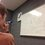# The Beauty of Math - Four Square Theorem

I came across this theorem one time and found it especially striking. I even told my friends who supposedly dislike math about it, and they were amazed, trying to calculate values for various numbers.

Lagrange's Four-Square Theorem states that any positive integer can be expressed as the sum of four perfect squares. Try this for numbers like 7, 31, 326. While we know of course of numbers that can be expressed as one square (perfect squares), we know less about numbers that can be expressed as the sum of two or three squares. (Note that 0 is a square). Try to work out this theorem for some other numbers? Can you find any that are the sum of only two squares or only three squares? Is there any pattern to these numbers?

While the proof of the theorem is beyond the scope of the Cosines Group, you can find the proof on the Wikipedia page with the same name.

As a Computer Science extension, can you create a code that will find the four squares that sum up to any given inputted number? Perhaps you can use this to locate any patterns in the system.

Feel free to post any solutions, ideas, questions, extensions, or code below.Note by Bob Krueger
6 years, 10 months ago

This discussion board is a place to discuss our Daily Challenges and the math and science related to those challenges. Explanations are more than just a solution — they should explain the steps and thinking strategies that you used to obtain the solution. Comments should further the discussion of math and science.

When posting on Brilliant:

• Use the emojis to react to an explanation, whether you're congratulating a job well done , or just really confused .
• Ask specific questions about the challenge or the steps in somebody's explanation. Well-posed questions can add a lot to the discussion, but posting "I don't understand!" doesn't help anyone.
• Try to contribute something new to the discussion, whether it is an extension, generalization or other idea related to the challenge.

MarkdownAppears as
*italics* or _italics_ italics
**bold** or __bold__ bold
- bulleted- list
• bulleted
• list
1. numbered2. list
1. numbered
2. list
Note: you must add a full line of space before and after lists for them to show up correctly
paragraph 1paragraph 2

paragraph 1

paragraph 2

[example link](https://brilliant.org)example link
> This is a quote
This is a quote
    # I indented these lines
# 4 spaces, and now they show
# up as a code block.

print "hello world"
# I indented these lines
# 4 spaces, and now they show
# up as a code block.

print "hello world"
MathAppears as
Remember to wrap math in $$ ... $$ or $ ... $ to ensure proper formatting.
2 \times 3 $2 \times 3$
2^{34} $2^{34}$
a_{i-1} $a_{i-1}$
\frac{2}{3} $\frac{2}{3}$
\sqrt{2} $\sqrt{2}$
\sum_{i=1}^3 $\sum_{i=1}^3$
\sin \theta $\sin \theta$
\boxed{123} $\boxed{123}$

Sort by:

- 6 years, 10 months ago

Sorry, started making a post and then realized that it was out of depth for #CosinesGroup, so I moved it.

Staff - 6 years, 10 months ago

superb

- 6 years, 10 months ago

Thank you!

- 6 years, 10 months ago

class Four_squares { public static void main(int n) { for(int a=0;a<=n;a++) for(int b=0;b<=n;b++) for(int c=0;c<=n;c++) for(int d=0;d<=n;d++) if(aa+bb+cc+dd==n) System.out.println(""+a+b+c+d); } }

- 6 years, 10 months ago

Short and simple. I like it. However, it's not efficient and its output is the cleanest. Are there any easy fixes to this?

- 6 years, 10 months ago

Very Ineffecient, sorry!

- 6 years, 10 months ago

This can be done in mathematica with this code: PowersRepresentations[n,4,2] Here is an interesting algorithm on it: http://www.alpertron.com.ar/4SQUARES.HTM

Source: http://www.mathisfunforum.com/viewtopic.php?id=19225

- 6 years, 10 months ago

By the way, the numbers that can be expressed as a sum of two squares have been completely characterized. Specifically, a number $n$ is a sum of two squares if and only if each prime divisor that is $3$ mod $4$ has an even exponent in the prime factorization of $n.$

- 6 years, 10 months ago

Just a random guess, you got this from PFTB? I got it from there :D

Well it is nice to prove this, here are some guidelines:

1. Prove that the product of two numbers that can both be represented as sums of two squares, can be represented as a sum of two squares.

2. Look at $\pmod 4$, obviously.

3. Another obvious fact but is used, the product of two squares is a square, and can be represented as a sum of two squares obviously.

4. You may use Fermat's Christmas Theorem (as it was in a letter dated on 1640's Christmas)..

This should be enough.

- 6 years, 10 months ago

Interesting!

- 6 years, 10 months ago

can you please tell what 'mod' means ? PLEASE !!!!!!!!!!!!!!!!!!!!!! I THINK YOU R GENIUS !!

- 6 years, 10 months ago

Considering numbers "mod 4" stands for looking at their remainders when divided by $4.$ Note that if you know the remainders, when divided by $4$, of any two numbers, you can calculate the remainder of their sum and product. For example, if $a$ has reminder $2$ and $b$ has remainder $3,$ their sum will always have remainder $1.$ You may want to take a look here: Modular Arithmetic

- 6 years, 10 months ago

THANKS

- 6 years, 10 months ago

Also, try the PowerRepresentations command in Mathematica

- 6 years, 7 months ago

This is so awesome.

- 6 years, 7 months ago

You can also check Goldbach's theorem which states that every positive even number can be expressed as the sum of two prime numbers

- 6 years, 10 months ago

This is actually not a theorem, but a conjecture, one of the most famous in Number Theory. There are some very interesting partial results, obtained using highly sophisticated methods.The Goldbach Conjecture Wikipedia page has a pretty accurate (though long) description.

- 6 years, 10 months ago